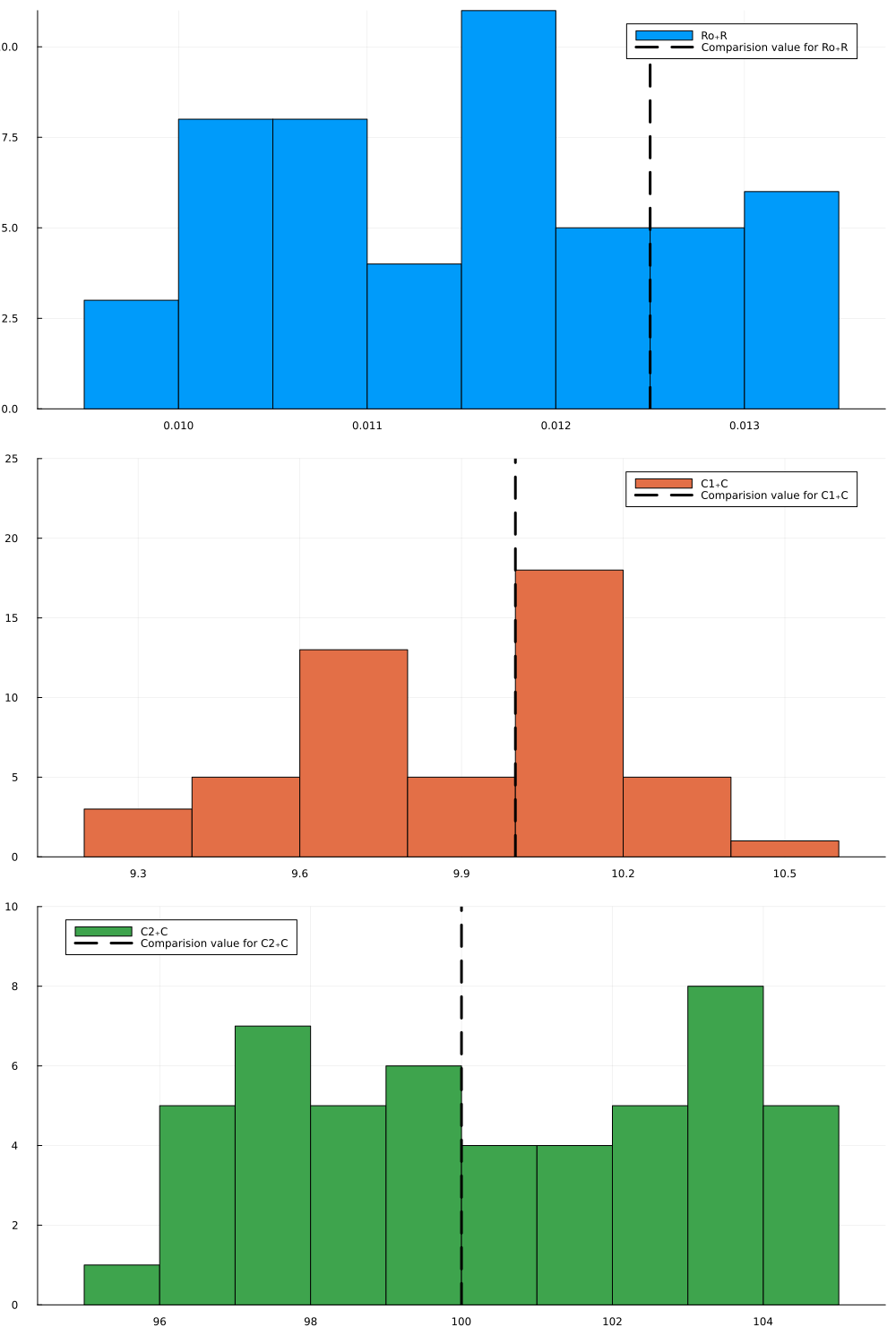# Uncertainty-Aware Parameter Estimation of an Acausal Circuit Model

Julia ModelSim Optimizer can be seamlessly integrated with custom components generated through the Modeling Toolkit Standard Library. The Chua Circuit electrical model is the first example we are exploring in this integration. Let us have a look at the sections below.

## Chua circuit model

Here we present the creation of a custom component is demonstrated via the Chua's circuit. The circuit is a simple circuit that shows chaotic behaviour. Except for a non-linear resistor every other component already is part of ModelingToolkitStandardLibrary.Electrical.

## Julia environment

# Packages used for this example
using JuliaSimModelOptimizer
using OrdinaryDiffEq
using ModelingToolkit
using DataFrames
using ModelingToolkitStandardLibrary.Electrical
using ModelingToolkitStandardLibrary.Electrical: OnePort
using Statistics
using StatsPlots
Plots.GRBackend()

## Model setup

The first step is to use the pre defined components defined in the Electrical Toolkit in the ModelingToolkit library. We can also define custom components such as the "Non linear resistor" as defined below. One advantage of using ModelingToolkit is being directly able to use custom components out of the box. We can define a Resistor component, a Capacitor component, an Inductor component etc. ModelingToolkit defined models can be seamlessly integrated with the solvers from DifferentialEquations.jl. In the code block below, the Rodas4 ODE solver has been used for the ODE forward simulation.

@parameters t

function NonlinearResistor(;name, Ga, Gb, Ve)
@named oneport = OnePort()
@unpack v, i = oneport
pars = @parameters Ga=Ga Gb=Gb Ve=Ve
eqs = [
i ~ ifelse(v < -Ve,
Gb*(v + Ve) - Ga*Ve,
ifelse(v > Ve,
Gb*(v - Ve) + Ga*Ve,
Ga*v,
),
)
]
extend(ODESystem(eqs, t, [], pars; name=name), oneport)
end

@named L = Inductor(L=18)
@named Ro = Resistor(R=12.5e-3)
@named G = Conductor(G=0.565)
@named C1 = Capacitor(C=10, v=4)
@named C2 = Capacitor(C=100)
@named Nr = NonlinearResistor(
Ga = -0.757576,
Gb = -0.409091,
Ve=1)
@named Gnd = Ground()

connections = [
connect(L.p, G.p)
connect(G.n, Nr.p)
connect(Nr.n, Gnd.g)
connect(C1.p, G.n)
connect(L.n, Ro.p)
connect(G.p, C2.p)
connect(C1.n, Gnd.g)
connect(C2.n, Gnd.g)
connect(Ro.n, Gnd.g)
]

@named model = ODESystem(connections, t, systems=[L, Ro, G, C1, C2, Nr, Gnd])

sys = structural_simplify(model)
prob = ODEProblem(sys, Pair[], (0, 5e4), saveat=100)
sol = solve(prob, Rodas4());
retcode: Success
Interpolation: 1st order linear
t: 501-element Vector{Float64}:
0.0
100.0
200.0
300.0
400.0
500.0
600.0
700.0
800.0
900.0
⋮
49200.0
49300.0
49400.0
49500.0
49600.0
49700.0
49800.0
49900.0
50000.0
u: 501-element Vector{Vector{Float64}}:
[0.0, 4.0, 0.0]
[3.2171613757658375, 4.504688408054067, 0.625256870863489]
[2.370736990059106, 1.8530602896688129, -0.8141498923948453]
[-2.021845767074865, -2.4582295433465484, -0.48409585703669034]
[-1.8158033058146492, -1.9204621805139885, 0.4320010322717699]
[0.28971709103022103, -1.2672826428324668, 0.042467461696191346]
[-1.784739221906928, -3.3417441203308402, -0.5786452915123017]
[-2.705336031251469, -2.688831668565868, 0.32006417304840756]
[0.29683551471395886, -0.6809830262589619, 0.38504365667955626]
[-0.46577119841656334, -2.487641278660753, -0.6085497744662725]
⋮
[0.7644351589247677, 2.660732388247788, 0.5232620259658282]
[2.981004247281878, 3.347389103978472, 0.049449704134730134]
[0.6607426512007414, 1.122464222690838, -0.5804376937152276]
[-0.2970445838426725, 1.6919238647215915, 0.3537315383076898]
[2.8794110786258025, 3.82442331318247, 0.45943821380264066]
[1.886752731554989, 1.7384073790613415, -0.6653181793954123]
[-1.1483490421258022, -0.7733221192256905, -0.18149685480097394]
[-0.8564107731918522, -1.724651342918787, 0.09225150802688872]
[-1.0602303912856623, -2.369253902004204, -0.18598971862727592]

## Creating the inverse problem

The next step is to define the inverse problem i.e specifying the parameters we want to optimize and the search space which we want to look for. The inverse problem consists of a collection of trials which define a multi-simulation optimization problem. The solution to an inverse problem are the "good parameters" which make the simulations simultaniously fit their respective data sufficiently well.

data = DataFrame(sol);

trial = Experiment(data, sys, tspan=(0, 5e4));

invprob = InverseProblem([trial], sys, [Ro.R => (9.5e-3, 13.5e-3), C1.C => (9, 11), C2.C => (95, 105)]);
InverseProblem for model with one experiment optimizing 3 parameters.


## Generating and visualizing the optimized parameters

In JuliaSimModelOptimizer, plausible populations are generated using the vpop function on an InverseProblem. The result of a vpop is a set of parameters which sufficiently fit all trials to their respective data simultaniously. In this example, we have 3 parameters which are simultaneously optimized: the resistance of the resistor Ro and the capacitances of the capacitors C1, C2.

Once the parameters have been optimized, we can use the statistical plotting libraries of Julia to generate histogram plots of the parameters. We can then compare the mean value of these histogram plots with the original parameter values.

ps = parametric_uq(invprob, StochGlobalOpt(maxiters=10), sample_size = 50)

plot(ps, layout=(3,1), show_comparision_value = true, max_freq = [10, 25, 10], legend = :best, size = (1000,1500))As we see above, the parameters recovered match the true parameters very well!